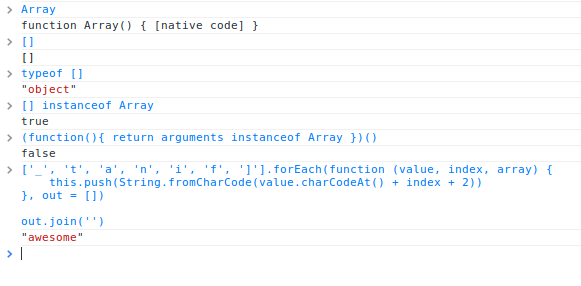17 January 2014

• 循环`.forEach`
• 断言`.some``.every`
• `.join``.concat`的区别
• 栈和队列`.pop``.push``.shift``.unshift`
• 模型映射`.map`
• 查询`.filter`
• 排序`.sort`
• 计算`.reduce``.reduceRight`
• 复制`.slice`
• 万能的`.splice`
• 查找`.indexOf`
• `in`操作符
• 走进`.reverse`## 循环`.forEach`

`forEach`方法需要一个回调函数，数组内的每个元素都会调用一次此方法，此方法需要三个参数如下：

• `value` 当前操作的数组元素
• 当前操作元素的数组索引
• `array` 当前数组的引用

``````['_', 't', 'a', 'n', 'i', 'f', ']'].forEach(function (value, index, array) {
this.push(String.fromCharCode(value.charCodeAt() + index + 2))
}, out = [])

out.join('')
// <- 'awesome'
``````

`.join`函数我将在下文提及，上面例子中，它将数组中的不同元素拼接在一起，类似于如下的效果：`out + '' + out + '' + out + '' + out[n]`

## 断言`.some`和`.every`

`some`将会给数组里的每一个元素执行一遍回调函数，直到有一个回调函数返回true位置。如果找到目标元素，`some`立即返回`true`，否则`some`返回`false`。回调函数只对已经指定值的数组索引执行；它不会对已删除的或未指定值的元素执行。

``````max = -Infinity
satisfied = [10, 12, 10, 8, 5, 23].some(function (value, index, array) {
if (value > max) max = value
return value < 10
})

console.log(max)
// <- 12

satisfied
// <- true
``````

## `.join`和`.concat`的区别

`.join`方法经常和`.concat`混淆。`.join`(分隔符)方法创建一个字符串，会将数组里面每个元素用分隔符连接。如果没有提供分隔符，默认的分隔符为“,”。`.concat`方法创建一个新数组，其是对原数组的浅拷贝（注意是浅拷贝哦）。

• `.concat` 的标志用法：`array.concat(val, val2, val3, valn)`
• `.concat` 返回一个新书组
• `array.concat()`没有参数的情况下，会返回原数组的浅拷贝

``````var a = { foo: 'bar' }
var b = [1, 2, 3, a]
var c = b.concat()

console.log(b === c)
// <- false

b === a && c === a
// <- true
``````

## 栈和队列`.pop`,`.push`,`.shift`和`.unshift`

`.pop`方法和`.push`成对使用，它返回数组的末尾元素并将元素从数组移除。如果数组为空，返回`void 0（undefined）`。使用`.push``.pop`我们能轻易模拟出LIFO（后进先出或先进后出）栈。

``````function Stack () {
this._stack = []
}

Stack.prototype.next = function () {
return this._stack.pop()
}

Stack.prototype.add = function () {
return this._stack.push.apply(this._stack, arguments)
}

stack = new Stack()
stack.add(1,2,3)

stack.next()
// <- 3
``````

``````function Queue () {
this._queue = []
}

Queue.prototype.next = function () {
return this._queue.shift()
}

Queue.prototype.add = function () {
return this._queue.unshift.apply(this._queue, arguments)
}

queue = new Queue()
queue.add(1,2,3)

queue.next()
// <- 1
``````

`.shift``.pop`能很容易遍历数组元素，并做一些操作。

``````list = [1,2,3,4,5,6,7,8,9,10]

while (item = list.shift()) {
console.log(item)
}

list
// <- []
``````

## 模型映射`.map`

`map` 方法会给原数组中的每个元素(必须有值)都调用一次 `callback` 函数`.callback` 每次执行后的返回值组合起来形成一个新数组。`callback`函数只会在有值的索引上被调用; 那些从来没被赋过值或者使用`delete`删除的索引则不会被调用。——MDN

`Array.prototype.map`方法和上面我们提到的`.forEach``.some``.every`有相同的参数：`.map(fn(value, index, array), thisArgument)`

``````values = [void 0, null, false, '']
values = void 0
result = values.map(function(value, index, array){
console.log(value)
return value
})

// <- [undefined, null, false, '', undefined × 3, undefined]
``````

`undefined × 3` 值解释`.map`不会在没被赋过值或者使用`delete`删除的索引上调用，但他们仍然被包含在结果数组中。`map`在遍历或改变数组方面非常有用，如下所示：

``````// 遍历
[1, '2', '30', '9'].map(function (value) {
return parseInt(value, 10)
})
// 1, 2, 30, 9

[97, 119, 101, 115, 111, 109, 101].map(String.fromCharCode).join('')
// <- 'awesome'

// 一个映射新对象的通用模式
items.map(function (item) {
return {
id: item.id,
name: computeName(item)
}
})
``````

## 查询`.filter`

`filter`对每个数组元素执行一次回调函数，并返回一个由回调函数返回`true`的元素 组成的新数组。回调函数只会对已经指定值的数组项调用。

``````[void 0, null, false, '', 1].filter(function (value) {
return value
})
// <- 

[void 0, null, false, '', 1].filter(function (value) {
return !value
})
// <- [void 0, null, false, '']
``````

## 排序`.sort`(比较函数)

• 如果`a``b`前，则返回值小于零
• 如果`a``b`是等价的，则返回值等于零
• 如果`a``b`后，则返回值大于零

``````[9,80,3,10,5,6].sort()
// <- [10, 3, 5, 6, 80, 9]

[9,80,3,10,5,6].sort(function (a, b) {
return a - b
})
// <- [3, 5, 6, 9, 10, 80]
``````

## 计算`.reduce`和`.reduceRight`

`previousValue`是最后被调用的回调函数的返回值，`initialValue`是开始时`previousValue`被初始化的值。`currentValue` 是当前被遍历的元素值，`index`是当前元素在数组中的索引值。`array`是对调用`.reduce`数组的简单引用。

``````Array.prototype.sum = function () {
return this.reduce(function (partial, value) {
return partial + value
}, 0)
};

[3,4,5,6,10].sum()
// <- 28
``````

``````function concat (input) {
return input.reduce(function (partial, value) {
if (partial) {
partial += ', '
}
return partial + value
}, '')
}

concat([
{ name: 'George' },
{ name: 'Sam' },
{ name: 'Pear' }
])
// <- 'George, Sam, Pear'
``````

## 复制`.slice`

`.concat`类似，调用`.slice`缺省参数时，返回原数组的浅拷贝。`slice`函数需要两个参数，一个是开始位置和一个结束位置。

`Array.prototype.slice`能被用来将类数组对象转换为真正的数组。

``````Array.prototype.slice.call({ 0: 'a', 1: 'b', length: 2 })
// <- ['a', 'b']
``````

``````Array.prototype.concat.call({ 0: 'a', 1: 'b', length: 2 })
// <- [{ 0: 'a', 1: 'b', length: 2 }]
``````

``````function format (text, bold) {
if (bold) {
text = '<b>' + text + '</b>'
}
var values = Array.prototype.slice.call(arguments, 2)

values.forEach(function (value) {
text = text.replace('%s', value)
})

return text
}

format('some%sthing%s %s', true, 'some', 'other', 'things')
// <- <b>somesomethingother things</b>
``````

## 万能的`.splice`

`.splice`是我最喜欢的原生数组函数之一。它允许你删除元素，插入新元素，或在同一位置同时进行上述操作，而只使用一个函数调用。注意和`.concat``.slice`不同的是`.splice`函数修改原数组。

``````var source = [1,2,3,8,8,8,8,8,9,10,11,12,13]
var spliced = source.splice(3, 4, 4, 5, 6, 7)

console.log(source)
// <- [1, 2, 3, 4, 5, 6, 7, 8, 9, 10, 11, 12 ,13]

spliced
// <- [8, 8, 8, 8]
``````

``````var source = [1,2,3,8,8,8,8,8,9,10,11,12,13]
var spliced = source.splice(9)

spliced.forEach(function (value) {
console.log('removed', value)
})
// <- removed 10
// <- removed 11
// <- removed 12
// <- removed 13

console.log(source)
// <- [1, 2, 3, 8, 8, 8, 8, 8, 9]
``````

## 查找`.indexOf`

``````var a = { foo: 'bar' }
var b = [a, 2]

console.log(b.indexOf(1))
// <- -1

console.log(b.indexOf({ foo: 'bar' }))
// <- -1

console.log(b.indexOf(a))
// <- 0

console.log(b.indexOf(a, 1))
// <- -1

b.indexOf(2, 1)
// <- 1
``````

## `in`操作符

``````var a = [1, 2, 5]

1 in a
// <- true, 但因为 2!

5 in a
// <- false
``````

``````var a = [3, 7, 6]

1 in a === !!a
// <- true
``````

`in`操作符类似于将键值转换为布尔值。`!!`表达式通常被开发者用来双重取非一个值（转化为布尔值）。实际上相当于强制转换为布尔值，任何为真的值被转为`true`，任何为假的值被转换为`false`

## 走进`.reverse`

``````var a = [1, 1, 7, 8]

a.reverse()
// [8, 7, 1, 1]
``````

## 译者注关注微信公众号 颜海镜赞赏支持 微信扫一扫